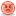PHP User Warning: fetch_template() calls should be replaced by the vB_Template class. Template name: bbcode_highlight in ..../includes/functions.php on line 4197

PHP User Warning: fetch_template() calls should be replaced by the vB_Template class. Template name: bbcode_highlight in ..../includes/functions.php on line 4197

PHP User Warning: fetch_template() calls should be replaced by the vB_Template class. Template name: bbcode_highlight in ..../includes/functions.php on line 4197

1. ##Answer not correct

Hi everyone,

I have the following code, can anyone tell me why it is returning zero, cos i cannot figure it out.
VB Code:
`Public A As Integer, B As IntegerPublic Formula As String Public Function FormulaBasher() As String        Dim FormulaScript As New ScriptControl        FormulaScript.Language = "VBScript"        A = 10    B = 5    Formula = "A+B"     FormulaBasher = CStr(FormulaScript.Eval(Formula))End Function`

Edit: Added [vbcode][/vbcode] tags for clairty. - HackReply With Quote

2. ## Re: Answer not correct

Your string Formula simply contains "A+B", rather than the actual values "10 + 5".

To put the values into the string you need to do this:
VB Code:
`Formula = A & "+" & B`Reply With Quote

3. ## Re: Answer not correct

How would the scriptcontrol know the value of A and B?
You tell it to Add A and B but will the control use the A and B declared in your module or make it's own, local variables A and B?Reply With Quote

4. ## Re: Answer not correct

I think that's still going to give a string, (10+5) as you're passing the plus-sign as a character. I reckon you have to run the Eval function on the same line as the assignment to Formula.
I don't understand the purpose of the code though. Why not just declare A and B as integers and be done with it?Reply With Quote

5. ## Re: Answer not correct

The Eval function of the scriptcontrol performs the calculations specified in strings (such as "(27+4) *2/3^4" if you like), and returns the value of that calculation.

jeroen79 is correct in that the scriptcontrol was creating its own variables A and B, which were both the default value (0).Reply With Quote

6. ## Re: Answer not correct

If the eval function is creating and using local variables, how can i get it to use the global variables???

I cannot create A and B as integers as i said before, because the formula could be ANYTHING!!! And can involve up to 14 variables!! A - L.

Thanks again!Reply With Quote

7. ## Re: Answer not correct

You cannot get Eval to use your variables, as it is completely separate from the scope of your program. You somehow need to place their values in the string as I did previously.

There is a simple solution though, you can use the Replace function to put the values into the string for you, eg:
VB Code:
`Public A As Integer, B As Integer, C as Integer, ...Public Formula As String Public Function FormulaBasher() As String        Dim FormulaScript As New ScriptControl        FormulaScript.Language = "VBScript"    A = 10    B = 5    C = 3    '...     Formula = "(A+B) * C + A"     'Replace the variables with their values.    Formula = Replace(Formula, "A", A)    Formula = Replace(Formula, "B", B)    Formula = Replace(Formula, "C", C)    '... 'temporary code, so you can see the changes have been made:MsgBox formula     FormulaBasher = CStr(FormulaScript.Eval(Formula))End Function`Reply With Quote

####Posting Permissions

• You may not post new threads
• You may not post replies
• You may not post attachments
• You may not edit your posts
•

Featured